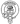# How to get a random element from an array in JavaScript

June 22, 2020

Often while generating test data for my databases I find myself needing a way in which to select a random value from an array in JavaScript. Sadly JavaScript doesn’t come with any built in utilities for this but we can build our own. Let’s first take a look at how to generate a random number between 0 and a maximum:

``````// Generates a number between 0 and 10
const random = Math.floor(Math.random() * 11)

console.log(random)

// 7``````

Now let’s generate a random number using this method with the maximum being our arrays length:

``````const roles = ['Admin', 'User', 'Guest', 'Restricted']

const randomRoleIndex = Math.floor(Math.random() * roles.length)

console.log(randomRoleIndex)

// 3``````

We can now get the associated element from the array using this index and also refactor a little bit to something more concise:

``````const roles = ['Admin', 'User', 'Guest', 'Restricted']

const randomRole = roles[Math.floor(Math.random() * roles.length)]

console.log(randomRole)

// Guest``````

If you plan on using this random function a lot throughout one of your projects you can add it to Array.prototype like so:

``````Array.prototype.sample = function() {
return this[Math.floor(Math.random() * this.length)]
}

const roles = ['Admin', 'User', 'Guest', 'Restricted']

const randomRole = roles.sample()

console.log(randomRole)